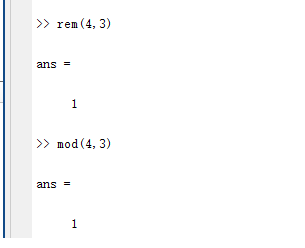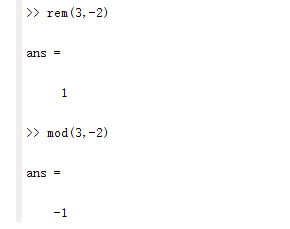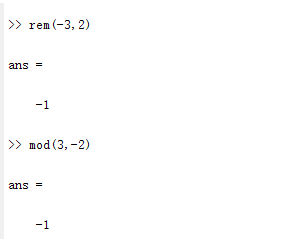• matlab mod和rem函数
千次阅读
2021-04-18 05:35:51

rem(x,y):求整除x/y的余数

mod(x,y):求模

rem(x,y)=x-y.*fix(x./y); (fix()向0取整)

mod(x,y)=x-y.*floor(x./y); (floor()向左取整)

如果x和y的符号相同(同为‘+’，同为‘-’)，那么rem(x,y)=mod(x,y)(正数与正数，负数与负数，取整结果两个函数效果一样)

如果x和y的符号相反，那么mod(x,y)=rem(x,y)+y(正数与负数的取整，看你希望得到什么结果就选择用什么函数)

当正数与负数取余时，当得到的余数结果的符号希望跟除数(x)一样，用rem()函数；当得到的余数结果的符号希望跟被除数(y)一样，用mod()函数

例如：

rem(3,2)=1;mod(3,2)=1;

rem(-3,-2)=-1;mod(-3,-2)=-1;

rem(3,-2)=1;mod(3,-2)=-1;

rem(-3,2)=-1;mod(-3,2)=1;

mod(3,2)=rem(3,2)=1;

mod(3,-2)=rem(3,-2)-2=1-2=-1;

mod(-3,2)=rem(-3,2)+2=-1+2=1;

更多相关内容
• 如果xy的符号相反，那么mod(x,y)=rem(x,y)+y(正数与负数的取整，看你希望得到什么结果就选择用什么函数) 当正数与负数取余时，当得到的余数结果的符号希望跟除数(x)一样，用rem()函数；当得到的余数结果的...
%求整数x/y的余数
rem(x,y)
%求模
mod(x,y)

如果x和y的符号相同（同为‘+’，同为‘-’），那么rem(x,y)=mod(x,y)（正数与正数，负数与负数，取整结果两个函数效果一样）如果x和y的符号相反，那么mod(x,y)=rem(x,y)+y(正数与负数的取整，看你希望得到什么结果就选择用什么函数)当正数与负数取余时，当得到的余数结果的符号希望跟除数(x)一样，用rem()函数；当得到的余数结果的符号希望跟被除数(y)一样，用mod()函数展开全文matlab
• 当xy的符号不同时，rem函数结果的符号x的一样，而mod和y一样。这是由于这两个函数的生成机制不同，rem函数采用fix函数，而mod函数采用了floor函数(这两个函数是用来取整的，fix函数向0方向舍入，floor函数向...

通常取模运算也叫取余运算，它们返回结果都是余数.rem和mod唯一的区别在于:

当x和y的正负号一样的时候，两个函数结果是等同的；当x和y的符号不同时，rem函数结果的符号和x的一样，而mod和y一样。

这是由于这两个函数的生成机制不同，rem函数采用fix函数，而mod函数采用了floor函数(这两个函数是用来取整的，fix函数向0方向舍入，floor函数向无穷小方向舍入)。

rem(x，y)命令返回的是x-n.*y，如果y不等于0，其中的n = fix(x./y)，而mod(x,y)返回的是x-n.*y，当y不等于0时，n=floor(x./y)

两个异号整数取模取值规律 (当是小数时也是这个运算规律，这一点好像与C语言的不太一样)

先将两个整数看作是正数，再作除法运算

①能整除时，其值为0

②不能整除时，其值=除数×(整商+1)-被除数

例：mod(36,-10)=-4

即：36除以10的整数商为3，加1后为4；其与除数之积为40；再与被数之差为(40-36=4)；取除数的符号。所以值为-4。

例：mod(9,1.2)=0.6;

例：

>> mod(5,2)

ans =1                   %“除数”是正，“余数”就是正

>> mod(-5,2)

ans =1

>> mod(5,-2)

ans =-1                  %“除数”是负，“余数‘就是负

>> mod(-5,-2)

ans =-1                  %用rem时，不管“除数”是正是负，“余数”的符号与“被除数”的符号相同

>> rem(5,2)

ans =1                   %“被除数”是正，“余数”就是正

>> rem(5,-2);

ans =1

>> rem(-5,2)

ans =-1                 %“被除数”是负，“余数”就是负

>> rem(-5,-2)

ans =-1

本文来源于网络：查看 >https://blog.csdn.net/u012939857/article/details/64141356

展开全文• mod函数用于取模运算。 语法形式为 M = mod(x,y)，这里的x,y可以是标量|向量|矩阵|多维数组。 M = mod(x,y)返回用 y除以 x后的余数，其中 x是被除数，y是除数。 计算方式为：当 y≠0 时，M=mod(x,y)= x - y.*...

### mod函数用于取模运算。

语法形式为 M = mod(x,y)，这里的x,y可以是标量 | 向量 | 矩阵 | 多维数组。

M = mod(x,y) 返回用 y 除以 x 后的余数，其中 x 是被除数，y 是除数。

计算方式为：当 y≠0 时，M=mod(x,y)= x - y.*floor(x./y)；当 y=0时，M=mod(x,0)=x，即mod函数遵从 mod(x,0) 返回 x 的约定。

### 举例如下：

1. 计算mod(20, 3)

20-3*floor(20/3)=20-3*floor(6.667)=20-3*6=20-18=2      注：floor函数是向下取整

MATLAB验证结果也是2。

2. 计算mod(22,-6)

22-(-6)*floor(22/(-6))=22-(-6)*floor(-3.6667)=22-(-6)*(-4)=22-24=-2

MATLAB验证结果也是-2。

### rem函数用于取余运算。

语法形式为 M = rem(x,y)，这里的x,y可以是标量 | 向量 | 矩阵 | 多维数组。

M = rem(x,y)返回用 y 除以 x 后的余数，其中 x 是被除数，y 是除数。

计算方式为：当 y≠0 时，M=rem(x,y)= x - y.*fix(x./y)；当y=0时，M=rem(x,0)=NaN，即rem 函数遵从 rem(x,0) 是 NaN

展开全文• M=mod(X,Y):M = mod(X,Y) if Y ~= 0, returns X - n.*Y where n = floor(X./Y). If Y is not an integer and the quotient X./Y is within roundoff error of an integer, then n is that integer. The inputs X and...
•matlab
• 当xy的符号不同时，rem函数结果的符号x的一样，而mod和y一样。 这是由于这两个函数的生成机制不同，rem函数采用fix函数，而mod函数采用了floor函数（这两个函数是用来取整的，fix函数向0方向舍入，floor函数向...
• rem(x,y):求整除x/y的余数mod(x... (floor()向左取整)如果xy的符号相同(同为‘+’，同为‘-’)，那么rem(x,y)=mod(x,y)(正数与正数，负数与负数，取整结果两个函数效果一样)如果xy的符号相反，那么mod(x,y)=rem(...
• ## 【 MATLAB 】rem函数介绍

万次阅读 多人点赞 2018-10-29 14:13:27
rem函数和mod函数很相似，二者认真看一个，另一个看一下区别即可。 mod函数介绍：【 MATLABmod 函数介绍 rem Remainder after division Syntax r = rem(a,b) Description r = rem(a,b) returns the ...
• rem mod （除后的余数） 语法： r=rem(a,b) ; b=mod(a,m) 其中 a 是被除数： 被除数，指定为标量、向量、矩阵或多维数组。a 必须是任意数值类型的实数值数组。数值输入 a b 必须具有相同的大小或兼容的大小...matlab
•   求余函数和求模函数有相同的地方但又不完全一致，主要的区别在于对负整数进行除法运算的操作不同。对于整数a，b来说，求余运算或求模运算的方法都是先求整数商c=a/b，再求余数或模r=a-c*b。求余运算在取c的值时...matlab
• 满意答案libaoxin2008推荐于 2019.10.24matlabrem()和mod()函数(2010-11-16 13:39:55) 转载rem(x,y):求整除x/y的余数mod(x,y):求模rem(x,y)=x-y.*fix(x./y); (fix()向0取整)mod(x,y)=x-y.*floor(x./y); (floor()向...
• (floor()向左取整,以数抽为准，朝负无穷方向取整)如果xy的符号相同(同为‘+’，同为‘-’)，那么rem(x,y)=mod(x,y)(正数与正数，负数与负数，取整结果两个函数效果一样)如果xy的符号相反，...
• (floor()向左取整,以数抽为准，朝负无穷方向取整)如果xy的符号相同(同为‘+’，同为‘-’)，那么rem(x,y)=mod(x,y)(正数与正数，负数与负数，取整结果两个函数效果一样)如果xy的符号相反，...
• ## matlab中rem与mod函数的区别

万次阅读 多人点赞 2015-10-10 08:50:53
语法格式： rem(x,y):求整除x/y的余数 mod(x,y):求模 ...rem(x,y)=x-y.*fix(x./y);...mod(x,y)=x-y.*floor(x./y);...如果xy的符号相同（同为‘+’，同为‘-’），那么rem(x,y)=mod(x,y)（正数与正数matlab rem
• mod ...mod 函数遵从 mod(a,0) 返回 a 的约定。 示例 标量被除后的余数 计算 23 对 5 取模: b = mod(23,5) b = 3 向量被除后的余数 计算一个整数向量除以除数 3 的余数: a = 1:5; m = 3; b = .matlab
• ## Matlab中的mod()函数

万次阅读 多人点赞 2020-05-05 19:20:58
mod 除后的余数（取模运算） 语法 b = mod(a,m) 说明 b = mod(a,m) 返回用 m 除以 a 后的余数...mod 函数遵从 mod(a,0) 返回 a 的约定。 示例 标量被除后的余数 计算 23 对 5 取模: b = mod(23,5) b = 3 向量被除后...matlab
• 当xy的符号不同时，rem函数结果的符号x的一样，而mod和y一样。  这是由于这两个函数的生成机制不同，rem函数采用fix函数，而mod函数采用了floor函数（这两个函数是用来取整的，fix函数向0方向舍入，floor函数向...
• matlab——fix，floor，ceil，round区别总结为：fix朝零方向取整，floor朝负无穷方向取整，ceil朝正无穷方向取整，round四舍五入到最近的整数(1)fix(x):截尾取整。如：>> fix([3.4 , -3.4])ans =3 -3(2)floor...
• ## Matlab中mod函数使用

千次阅读 2020-12-07 13:41:11
mod rem 的区别 同余关系 三.示例 1.标量被除后的余数 2.向量被除后的余数 3.正值负值被除后的余数 4.用负除数除后的余数 5.浮点值被除后的余数 mod函数是两个数相除后的余数（取模运算）。 一.语法 ...matlab
• mod的结果取决于除数的符号，如果除数是正，则余数也为正；反之则余数为负。 mod(1,4)=1; mod(-2,3)=1; mod(2,-3)=-1; rem的结果取决于被除数的符号，如果被除数为正，则余数也为正；反之则余数为负。 rem(1,4)=1; ...
• rem和mod是求余的，但是算法不同，差异在哪呢？   就在于分别使用了fixfloor, 函数fixfloor都是圆整用的，fix是向0的方向圆整，如fix(-1.5)=-1,fix(1.5)=1;而floor是向下圆整，如floor(-1.5)=-2,floor(1.5...MATLAB
• 学习Matlab的数学函数时，教程中提到取模（mod）与取余（rem）是不同的matlab
• 1、xor()括号里的两个命令当且仅当只有一个逻辑运算正确的时候，返回逻辑值为1。 2、运算优先级。 容易错的有：逻辑运算在加减法后面， ...对于舍入误差在Matlab中的解决办法，通常是：判断两个数的差的绝对值，若小于matlab...